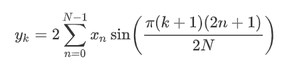Skip to content
Related Articles
Python – scipy.fft.dst() method
• Last Updated : 01 Oct, 2020

With the help of scipy.fft.dst() method, we can compute the discrete sine transform by selecting different types of sequences and return the transformed array by using this method.Syntax :

```scipy.fft.dst(x, type=2)
```

Return value: It will return the transformed array.

Example #1: In this example, we can see that by using scipy.fft.dst() method, we are able to get the discrete sine transform by selecting different types of sequences by default it’s 2.

## Python3

 `# import scipy``from` `scipy ``import` `fft`` ` `# Using scipy.fft.dst() method``gfg ``=` `fft.dst([``1``, ``2``, ``3``, ``4``])`` ` `print``(gfg)`

Output :

```[13.06562965 -5.65685425  5.41196100 -4.00000000]
```

Example #2 :

## Python3

 `# import scipy``from` `scipy ``import` `fft`` ` `# Using scipy.fft.dst() method``gfg ``=` `fft.dst([``-``6``, ``5``, ``-``4``, ``3``, ``-``2``, ``1``], ``3``)`` ` `print``(gfg)`

Output :

```[ -1.43023367  -2.3137085   -6.16568427  -7.77337942 -20.3137085
-23.82253852]
```

Attention geek! Strengthen your foundations with the Python Programming Foundation Course and learn the basics.

To begin with, your interview preparations Enhance your Data Structures concepts with the Python DS Course. And to begin with your Machine Learning Journey, join the Machine Learning – Basic Level Course

My Personal Notes arrow_drop_up